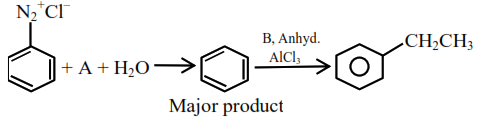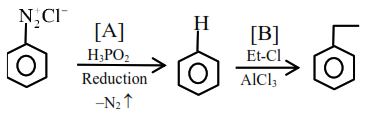# Solve thisQuestion:In the chemical reactions given above $\mathrm{A}$ and $\mathrm{B}$ respectively are :

1. $\mathrm{H}_{3} \mathrm{PO}_{2}$ and $\mathrm{CH}_{3} \mathrm{CH}_{2} \mathrm{Cl}$

2. $\mathrm{CH}_{3} \mathrm{CH}_{2} \mathrm{OH}$ and $\mathrm{H}_{3} \mathrm{PO}_{2}$

3. $\mathrm{H}_{3} \mathrm{PO}_{2}$ and $\mathrm{CH}_{3} \mathrm{CH}_{2} \mathrm{OH}$

4. $\mathrm{CH}_{3} \mathrm{CH}_{2} \mathrm{Cl}$ and $\mathrm{H}_{3} \mathrm{PO}_{2}$

Correct Option: 1

Solution: